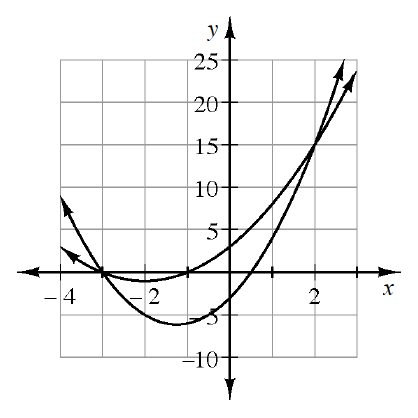### Home > INT3 > Chapter 9 > Lesson 9.1.4 > Problem9-50

9-50.

The graphs of $f\left(x\right) = 2x^{2} + 5x – 3$ and $g\left(x\right) = x^{2} + 4x + 3$ are shown at right. Use the graphs to solve:

1. $f\left(x\right) = g\left(x\right)$

When the equations of graphs are set equal to each other, their points overlap.

2. $f(x)>g(x)$

In terms of $x$, where on the graph do the $y$-values of $f\left(x\right)$ exceed those of $g\left(x\right)$?

$x<−3$ and $x>2$